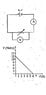# Non-ideal voltmeters and ammeters

• Eitan Levy

## Homework Statement

Someone did an experiment with an ideal voltmeter and an ideal ampermeter using the circuit in the picture, and got the graph in the picture.
-We know use an unideal voltmeter, how will the graph change?
-We know use an unideal ampermeter (the voltmeter is ideal), how will the graph change?

V=IR
u=V-Ir

## The Attempt at a Solution

The graph basically displays u=ε-Ir.
I figured that if the voltmeter is not ideal, the same current measured by the ampermeter will be "shared" by the voltmeter and the resistor, causing a higher value for the same current. In the answers they say it won't change.
Then, I figured that if the ampermeter is not ideal, the value will decrease quicker, becuase now u=ε-Ir-IR, this one doesn't have an answer.
Where is my mistake? Am I right?

#### Attachments

•Screenshot_20180529-184928.jpg
12.3 KB · Views: 516

What you should do is redraw the circuit replacing...

a) the symbol for a battery with symbols representing a non ideal battery. In this case you have enough info to calculate r but it's not needed for the problem.

And

b) the symbol for a volt meter with symbols representing a non ideal voltmeter.

What you should do is redraw the circuit replacing...

a) the symbol for a battery with symbols representing a non ideal battery. In this case you have enough info to calculate r but it's not needed for the problem.

And

b) the symbol for a volt meter with symbols representing a non ideal voltmeter.

Hi, this is the only way we learned to draw them.

Ok well...

A non ideal battery can be drawn as an ideal battery with a resistor in series.

A non ideal volt meter can be drawn as an ideal volt meter with a resistor in parallel.

Forum rules don't allow us to just give you the answer but we can help you work it out.

Forum rules don't allow us to just give you the answer but we can help you work it out.
I am aware of the rules, but I would like to at least know what is wrong with my explanation so I could come up with a new one.

Ok..

I figured that if the voltmeter is not ideal, the same current measured by the ampermeter will be "shared" by the voltmeter and the resistor, causing a higher value for the same current. In the answers they say it won't change.

The current won't be the same because the volt meter is non ideal. The answer depends on how non ideal it is. You can get the book answer if you make some assumptions. Compare the value of the battery resistance r with likely values for the resistance of a non ideal voltmeter.

Then, I figured that if the ampermeter is not ideal, the value will decrease quicker, becuase now u=ε-Ir-IR, this one doesn't have an answer.
Where is my mistake? Am I right?

Correct.

Ok..

The current won't be the same because the volt meter is non ideal. The answer depends on how non ideal it is. You can get the book answer if you make some assumptions. Compare the value of the battery resistance r with likely values for the resistance of a non ideal voltmeter.

Correct.
What I don't understand is, why doesn't this happen:

#### Attachments

All should become clear if you replace the voltmeter with the model for a non ideal voltmeter.

Hint: Two resistors in parallel always have a combined resistance lower than either of the individual resistors. So the current in the circuit will increase.

Hint: Two resistors in parallel always have a combined resistance lower than either of the individual resistors. So the current in the circuit will increase.
It will, but I am talking about how different would a certain point be on the graph for the SAME current. This is what the graph shows, no?

Ok I missunderstood what you meant by same current.

If you replace the ideal volt meter with a non ideal meter AND readjust the variable resistor so the current stays the same THEN the voltage drop through the battery resistance r will be the same and so the voltmeter reads the same.

It's worth thinking about the case when the current = 0. With an ideal meter this occurs when the variable resistor has infinite resistance. With the non ideal meter the total resistance can't be infinite because of the meter resistance. If you assume the meter resistance >> than r this can be ignored. But this isn't always true. For example if the battery was actually a capacitor r would be quite large.